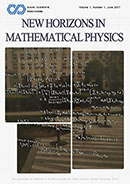Isaac Scientific Publishing
New Horizons in Mathematical Physics
•• New Horizons in Mathematical Physics (NHMP) is dedicated to publishing high-quality articles on current developments of mathematical methods for application to problems in physics and for the formulation of physical theories, as well as the feedback to pure and applied mathematics.

The journal aims at the fruitful dialogue between physics and mathematics, in tradition and new guises.

It covers quantum field theory, general relativity and gravitation, string theory, relativistic theories, quantum mechanics, quantum information and computation, classical mechanics, fluids, statistical physics, and other active areas of mathematical physics, and their interplay with partial differential equations, representation theory, finite and Lie groups, combinatorics, algebraic and differential geometry, topology, and number theory.

Editor-in-Chief: Prof. Yang-Hui He, City, University of London, UK
ISSN: 2521-7410 (Online)
ISSN: 2521-7402 (Print)

Contact
Email: nhmp@isaacpub.org